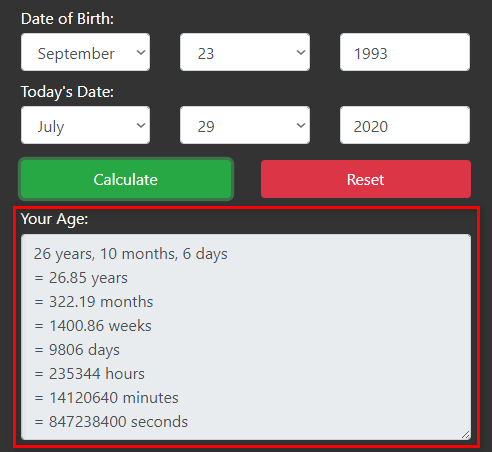# Age Calculator

Age Calculator allows you to find your age in years, months, weeks, days, hours, minutes, and even seconds.

Age Calculator is a free online tool where you can determine your exact age in years, months, and days. Also, it gives very accurate age results. Just input your date of birth and today's date and press the "Calculate" button. As a result, you will get your age within a second.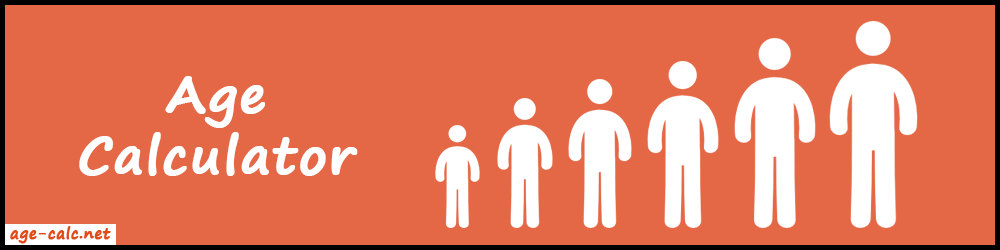#### Why developed Age Calculator?

Age is a very important part of our life. Also, it's used in many places. Generally, we are using it in identity proof certificates. Such as Passport, Driving License, Library Card, etc.

So, when we apply for a passport or driving license then there is always a blank field that asks us to enter our age. Sometimes we need to enter our exact age(in years, months, and days) on the form. So, it's very hard to figure it out manually. That's why we created an Age Calculator to know your exact age online.

It's a great tool that lets you know how old you are. Also, it's completely free to use with a super simple process. Moreover, you can use this tool to find ages for different dates too. So, use this free app and compute your exact age quickly.

### How to Calculate Age?

Most importantly, the age calculator takes two inputs from users.

1. Date of Birth
2. Today's Date

So, to calculate age manually, we have to subtract today's date with birth date. As a result, we get duration between two dates and that's your "Age".

Let's take some examples to understand it deeply. Also, in these examples, we will calculate only the years, months, and days.

##### Example 1:

Suppose Jason's date of birth is 05 January, 1990. Today's date is 10 September, 2019. Find out how old Jason is?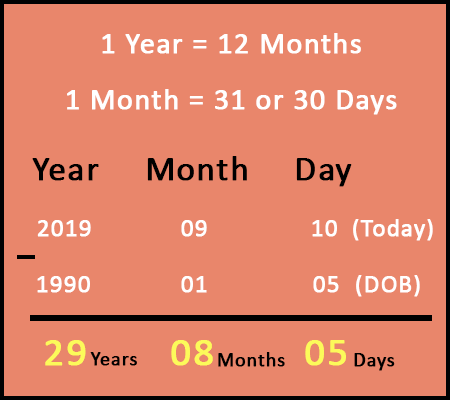So, Jason is 29 years, 8 months, and 5 days old.

Now the question arises, what if today's date of month is smaller than DOB's month?

So, let's take one example for that type of case.

##### Example 2:

Find the age for:

• DOB = 10 August, 1994
• Today's Date = 24 March, 2020As you can see above, 03 is less than 08.

So, in this case we will take 1 year = 12 months from 2020 and add it to 03 months.

Now, months will be 03 + 12 = 15 and year will be 2020 - 1 = 2019.

After subtraction, we get 25 years, 7 months, and 14 days.

What if today's date of month and day both are smaller than DOB's month and day?

You will get this question's answer in next example.

##### Example 3:

Let's take

• DOB = 15 May, 1985
• Today's Date = 05 February, 2018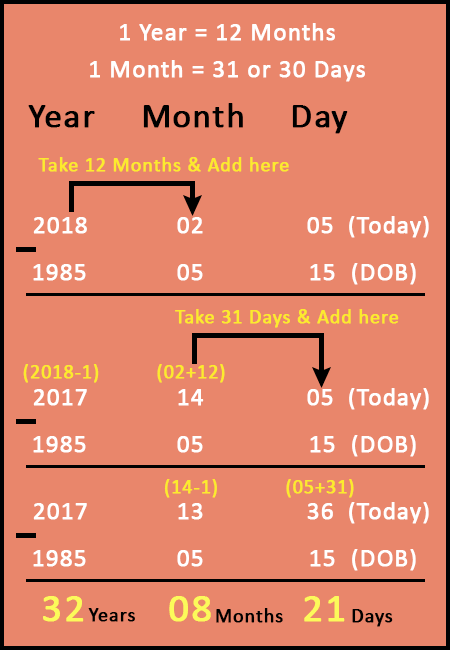In this case, Month 02 is less than 05 and Day 05 is less than 15.

So, firstly, we will take 1 year = 12 months from 2018 and add it to 02 months.

As a result, Months = 02 + 12 = 14, and Year = 2018 - 1 = 2017.

After that, we will take 1 month = 31 days from 14 months and add it to 05 days.

Therefore, Days = 05 + 31 = 36, and Months = 14 - 1 = 13.

Finally, Age = 32 years, 08 months, and 21 days.

#### Features of Age Calculator

There are so many nice features that make our tool more convenient and unique from other online resources.

• Easy to Use: The user interface is very simple and user-friendly. Anyone can easily walk through the calculation process.
• Handy Calculator: We have made it mobile and iPad responsive. So, you can use it on your handy devices like mobile and iPad.
• Simple three-step process: Enter the born date and today's date and press the "Calculate" button. So, you can calculate your age in just a few keystrokes.
• Multiple Outputs: It will not show your age only in years. You can find your age in years, months, weeks, days, hours, minutes, and even seconds.
• No Manual Calculation: Forget about using a pen-paper to know your age. Also, it's very time consuming and there is a chance to get false result. No need to do the manual calculation when you are using our tool.
• Fast Results: Know your age within a second using our tool. Because it works on high standard javascript coding language. So, it gives results in less than a second. Also, our tool is very lightweight and works on low internet speed too.
• 100% Accurate Results: Our tool gives 100% accurate results. But the input data must be correct to get error-free output.

### How to use Age Calculator?

Firstly, open the Age calculator on your device. After that, you can see some input boxes at the top of the page.

There are just three simple steps that you need to follow.

1. Enter Date of Birth
2. Enter Today's Date or Age at the Date of

Let's understand above all steps one by one.

##### 1. Enter Date of Birth

Firstly, you need to enter your birth date. Also, you can use the drop-down options to select month and day. In addition, you can enter the year using the keyboard.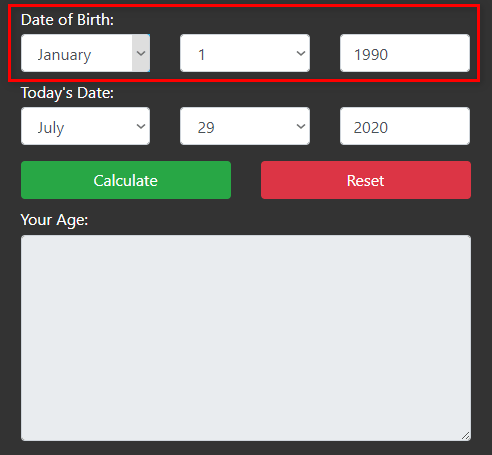##### 2. Enter Today's Date or Age at the Date of

This is the second step that you need to follow. In this step, you have to enter today's date. By default, it is selected as today's date. So, you can ignore it. But if you want to find the age for a different date, then you can enter other dates manually.